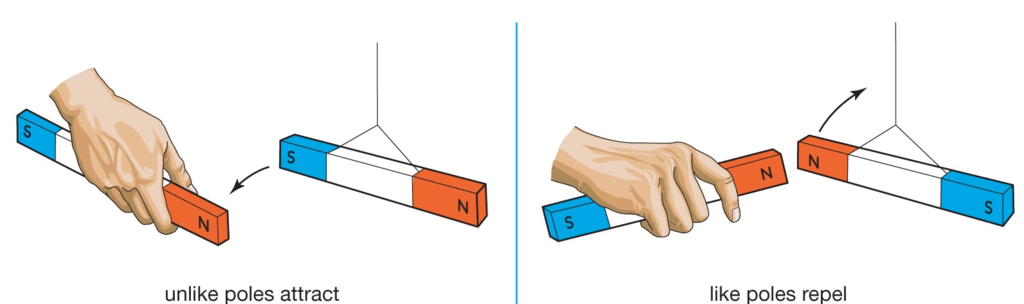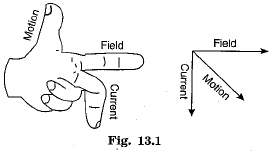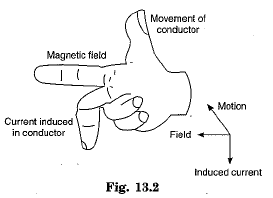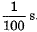Courses

# Overview of Magnetic Effects of Electric Current Class 10 Notes | EduRev

## Class 10 : Overview of Magnetic Effects of Electric Current Class 10 Notes | EduRev

The document Overview of Magnetic Effects of Electric Current Class 10 Notes | EduRev is a part of the Class 10 Course Science Class 10.
All you need of Class 10 at this link: Class 10

1. A magnet attracts magnetic substances, e.g., iron, steel etc., towards it. If a magnetic needle is freely suspended, it comes to rest in north-south direction. The end pointing towards north is called north pole and the other end pointing towards south is called south pole.

2. Two poles of a magnet cannot be separated and magnetic poles are always found in pair.

3. Like magnetic poles repel, while unlike magnetic poles attract each other.4. The region around a magnet, in which the force (influence) of magnet can be detected, is called its magnetic field. The direction of a magnetic field at a point is the direction in which the north pole of a compass needle points. The magnetic field can be represented by magnetic field lines.

5. A magnetic field line at any point gives the direction of magnetic field. Tb specify the direction of magnetic field arrow marks are marked on the magnetic field lines. The relative strength of the magnetic field is shown by the degree of closeness of the field lines.
The field is stronger, where the field lines are more crowded.
No two magnetic field lines can ever intersect at any point. If they do so then there will be two possible directions of magnetic field at the point of intersection, which is not possible.
Outside a magnet, magnetic field lines start from north pole and end at the south pole of a magnet. However, inside the magnet the field lines are directed from south pole to north pole, Thus, magnetic field lines form closed paths.

6. Oersted experimentally proved that magnetic field is produced around a conductor when an electric current is passed through it.
The magnitude of the magnetic field produced at a given point near a current-carrying straight conductor is directly proportional to the current passing in the conductor. Moreover, magnetic field decreases as the distance of the point from the conducting wire increases.

7. The direction of magnetic field associated with a current-carrying wire can be easily found by applying the “right-hand thumb rule”. According to this rule, hold the current- carrying wire in your right-hand such that the thumb is stretched along the direction of current, then, the fingers will wrap around the wire in the direction of the magnetic field.

8. Magnetic field is developed around a current-carrying circular wire loop. It is found that the magnetic field at the centre of wire loop is proportional to the value of current and inversely proportional to the radius of loop. Moreover, if we have a circular coil of n turns, the magnetic field will be n times of the magnetic field due to a single current loop.

9. Direction of magnetic field due to circular current loop can be found by right-hand rule. Magnetic field lines are circular closed loops around the conductor. As we go away from the loop, these closed loops become bigger and bigger. At the centre of loop, the magnetic field appears as straight line.

10. A solenoid is a coil of a large number of circular turns of enamelled copper wire wrapped on a long cardboard cylindrical base. On passing electric current through a solenoid, a magnetic field is developed along the axis of solenoid.
The magnetic field due to a current-carrying solenoid coil is very much similar to that of a bar magnet. One end of the solenoid coil acts as the magnetic north pole and the other end acts as the magnetic south pole. The field lines inside the solenoid are parallel straight lines along the axis of solenoid. Moreover, the magnetic field is a uniform field.

11. A strong magnetic field produced by passing a current through a solenoid coil is used to magnetise a piece of iron or any other magnetic material when the piece is placed within the solenoid coil. This type of magnet is known as an electromagnet.

12. Ampere proved that a current-carrying conductor placed in an external magnetic field experiences a mechanical force due to the magnetic field.
The force due to magnetic field depends on the length of the conductor, the amount of current flowing through it and the strength of magnetic field. Moreover, the force depends on relative directions of current and magnetic field. The force is maximum when the direction of current is at right angles to the direction of the magnetic field.

13. Direction of force acting on a current-carrying conductor due to a magnetic field can be easily found by applying Fleming’s left-hand rule.
Fleming’s left-hand rule states that stretch the forefinger, the central finger and the thumb of your left-hand in mutually perpendicular directions. If the forefinger shows the direction of the magnetic field and the central finger that of the current, then the thumb will point towards the direction of motion of the conductor (i.e., the thumb will point in the direction of force F)14. Electric motor is a device that converts electrical energy into mechanical energy.
An electric motor works on the principle that a coil carrying current, when placed in a uniform magnetic field, experiences a force whose direction is given by Fleming’s left- hand rule. Electric motors are used in electric fans, refrigerators, mixers, washing machines, computers and practically all machines operating on electrical power.

15. Faraday demonstrated that motion of a magnet with respect to a coil can induce a potential difference between the ends of coil which, in turn, sets up an induced electric current in the coil. In general, an induced current is set up in a closed coil whenever magnetic field near it is changing. It is known as the phenomenon of “electromagnetic induction”. Direction of induced current is given by Fleming’s right-hand rule.

16. Fleming’s right-hand rule states that hold the forefinger, the central finger and the thumb of the right-hand mutually perpendicular to each other such that the forefinger indicates the direction of magnetic field and the thumb is in the direction of motion of a conductor. Then, the central finger shows the direction of current induced in the conductor.

17. A galvanometer is an instrument which can detect the presence of current in a circuit.

18. Induced current due to electromagnetic induction can be obtained in a coil either by changing the magnetic field around it or by moving the coil in a magnetic field. However, it is convenient to move the coil in a magnetic field.

19. An electric generator (or dynamo) is a device used for generating electric current by converting mechanical energy into electrical energy.
An electric generator works on the principle of electromagnetic induction. Mechanical energy is used to rotate a conductor or a coil in a magnetic field so as to produce electricity. Direction of induced current is given by Fleming’s right-hand rule.

20. Electric current, whose magnitude as well as direction remains throughout constant (or same), is called a direct current (D.C.). Current supplied by a battery is a direct current.

21. A current, whose magnitude continuously varies with time and which changes its direction after equal intervals of time, is called an alternating current (A.C.). Current produced by an a.c. generator and the current being supplied in our houses is A.C. In India, the frequency of A.C. is 50 Hz. It means that the A.C. changes its direction after every22. In domestic electric circuits, all points are connected in parallel so that (a) voltage across each appliance is same, (b) separate switch can be put in the circuit of each appliance and (c) short-circuiting of one appliance does not affect the working of other appliances.

23. The domestic supply circuit consists of three types of wires, namely, (i) live wire having red insulation, (ii) neutral wire having black insulation, and (iii) earth wire having green coloured insulation. Switches are provided in live wire before the appliance. Generally, two separate circuits are used in domestic supply, one of 15 A for appliances of high power rating, e.g., electric stove, geyser, cooler, air conditioner, fridge etc., and the other of 5 A ratings for bulbs, tubes and fans.
The watt-hour meter (or electricity meter) measures the total amount of electrical energy consumed in a house and the distribution box contains main switches and fuses for each circuit.

24. Short-circuiting takes place in domestic power supply if the live wire and the neutral wire come into direct contact, without any load in between, either due to some fault in an electric appliance or due to damage to insulation of line wire.

25. Overloading of an electric circuit is caused by connecting too many appliances to a single socket.

26. Fuse is the most important safety device used in domestic power supply circuits. It is used to protect the circuits in case of either short-circuiting or overloading of the circuits. Fuse wire is prepared from a metal whose resistivity is high but whose melting point is low. The fuse wire melts due to overheating when current flowing through a circuit rises beyond a safe limit. As a result the current flow in the circuit stops and no damage is caused to the circuit.

Offer running on EduRev: Apply code STAYHOME200 to get INR 200 off on our premium plan EduRev Infinity!

## Science Class 10

85 videos|255 docs|129 tests

,

,

,

,

,

,

,

,

,

,

,

,

,

,

,

,

,

,

,

,

,

;